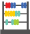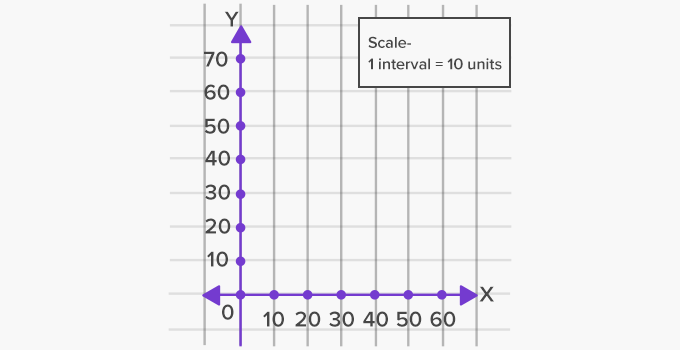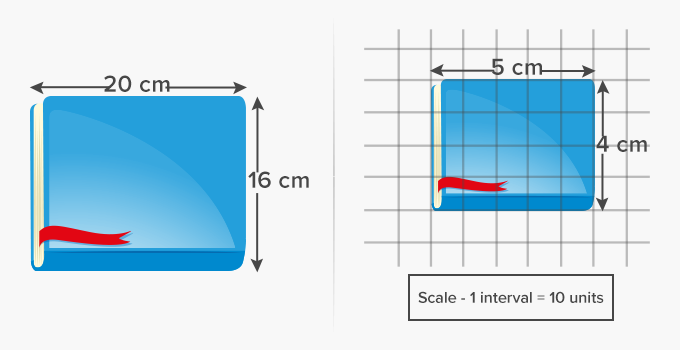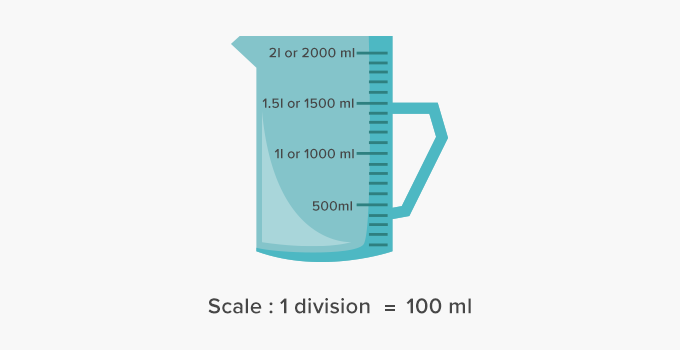# Scale - Definition with Examples

The Complete K-5 Math Learning Program Built for Your Child

• 30 Million Kids

Loved by kids and parent worldwide

• 50,000 Schools

Trusted by teachers across schools

• Comprehensive Curriculum

Aligned to Common Core

##Let's learn!

What is a scale?
In math, a scale in graphs can be defined as the system of marks at fixed intervals, which define the relation between the units being used and their representation on the graph.

Here, for instance, the scale of the graph is 1 interval being equal to 10 units.Here, the actual length of the book is represented on a graph using the scale, 1 interval is equal to 4 units.Here, we can see a scale on the measuring jug. Each small interval or division measures 100 ml.Fun Facts A ruler is often called a scale.  The measuring tool used for determining the weight of a body is also called a scale. Digital scales are the most commonly available scales around us.

##Let's do it!

Ask your child to measure the length and width of an object and then, represent it on a graph using an appropriate scale.

##Related math vocabulary

Won Numerous Awards & Honors